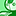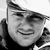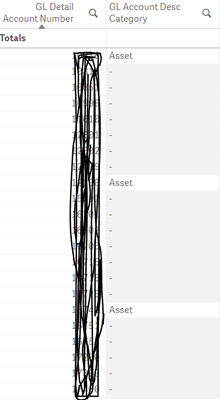Announcements
Applications are open for the 2024 Qlik Luminary Program. Apply by December 15 here.
cancel
Showing results for
Did you mean:Partner - Newbie

## Multiple If Statements with OR condition

Hi All,

I'm trying to group account numbers into categories so I can see which accounts belong in Assets, Liability, etc.

I used the script below to do this but something went wrong between Revenue and Expense. All the account numbers that should be in Expense are also considered Revenue.

If(Left(bga_bsc_gl_acct, 1) = 1, 'Asset',
If(Left(bga_bsc_gl_acct, 1) = 2,'Liability',
If(Left(bga_bsc_gl_acct,1) = 3,'Equity',
If(Left(bga_bsc_gl_acct,2) = 41 or 42 or 76,'Revenue',
If(Left(bga_bsc_gl_acct,2) = 47 or 48 or 49 or 51 or 52 or 53 or 66 or 83 or 86,'Expense','Other'))))) as [GL Detail Account Desc Category]

Any help or insight on this issue would be greatly appreciated!

Thanks~

Labels (2)

• ### Script

1 Solution

Accepted SolutionsMVP

Try to change your expression to a pick match function instead. (I've left out the Expense-part, but hopefully you understand the logic)

`= pick(     wildmatch( bga_bsc_gl_acct,'1*','2*','3*','41*','42*','76*','*'),    'Asset', 'Liability', 'Equity', 'Revenue','Revenue','Revenue','Other'      )`

If you dont want to change then you will need to change your logic from equal sign and ORs to match()

`if(match(Left(bga_bsc_gl_acct,2),41,42,76),'Revenue')`
4 RepliesMVP

Try to change your expression to a pick match function instead. (I've left out the Expense-part, but hopefully you understand the logic)

`= pick(     wildmatch( bga_bsc_gl_acct,'1*','2*','3*','41*','42*','76*','*'),    'Asset', 'Liability', 'Equity', 'Revenue','Revenue','Revenue','Other'      )`

If you dont want to change then you will need to change your logic from equal sign and ORs to match()

`if(match(Left(bga_bsc_gl_acct,2),41,42,76),'Revenue')`Partner - Newbie
Author

Thank you! This works although I do have a question-

When I used this approach as a dimension in my table I noticed large gaps where there should be a category listed and Revenue and Equity do not appear in the search bar. Do you have any idea why this could occur?

Just a note, Revenue and Equity both balance out to \$0 while the other categories have values but I still expected to see their account information if I were to search for it.MVP

It is hard to help with such little information, but I'll give it a shot.

If the GL Detail Account Number  is an key dimension. In other words a dimension that links two or more tables together.

Then my guess would be that the blank accounts are not found in the table where you are doing the Category classifications. E.g. you are doing the classifications on the dimension table, but have more accounts in the transaction table.Partner - Newbie
Author
Yes, it is a key dimension! In this situation, am I better off doing some kind of join to bring in those accounts? I moved my Category script to the transaction table but I'm not sure if this is the correct.

Thank you!Tags
Community Browser